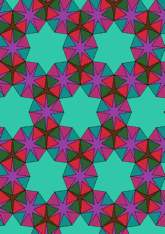# Derived from figure 2.5.4 (k) by adding trianglesdata146/F424D

## Geometry

• The symmetry group of the tiling is *632 (p6m).
• All the internal angles of the constituent polygons are a multiple of 15°.
• Contains four equilateral triangles.
• Contains one regular three-pointed star polygon with vertex angle of 15°.
• Contains one regular 6-pointed star polygon with vertex angle of 75°.
• Contains one regular 8-pointed star polygon with vertex angle of 15°.
• The tiling satisfies the two-colour condition.
• The tiling is edge-to-edge.
• As drawn, contains about 449 polygons.

## References

Publications referenced:
1. of B A Wichmann. The World of Patterns, World Scientific, 2001. ISBN 981024619. [wich2] {Only new patterns ascribed to this.}

v53